# NCERT Solutions for Class 10 Maths Chapter 1 - Real Numbers

Share

Goprep provides students with NCERT Mathematics Solutions for Class 10th Chapter 1- Real Numbers to assist students in understanding the concepts of the topics and solving the questions of the NCERT textbook in a simple and quick way. These Solutions are based on the latest CBSE syllabus and help students in attaining the problem-solving skills. Having online access of the same, these Solutions simplify the complex fundamentals of the topics in an efficient way.

The Chapter begins with a discussion of the two important properties of Positive Integers, Revisiting Irrational Numbers, Fundamental Theorem of Arithmetic, Multiplication of Positive Integers, etc. With the help of these Solutions, you can clear your concepts which are helpful in later grades as well. These guided NCERT Solutions are sure to help students in performing and scoring more marks in the examinations by making their foundation strong of the subject.

## NCERT Solutions for Class 10 Maths Chapter 1 - Real Numbers

Exercise 1.1
• Exercise 1.1
• Exercise 1.2
• Exercise 1.3
• Exercise 1.4
Class 10th|NCERT - MathematicsChapter 1 - Real Numbers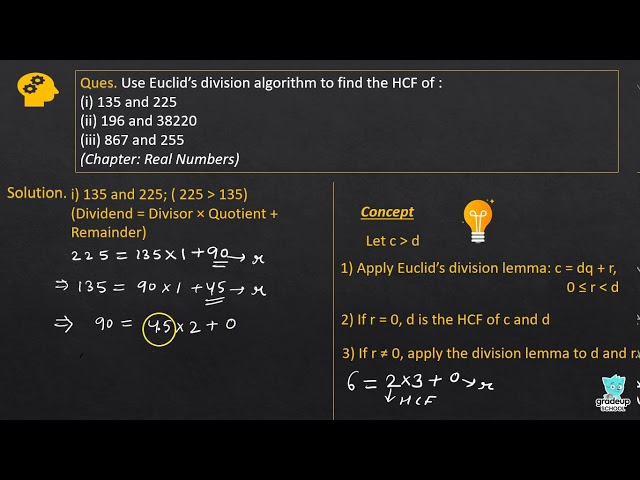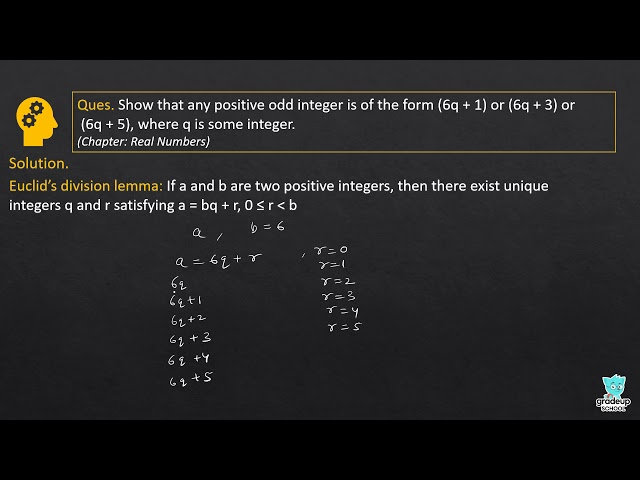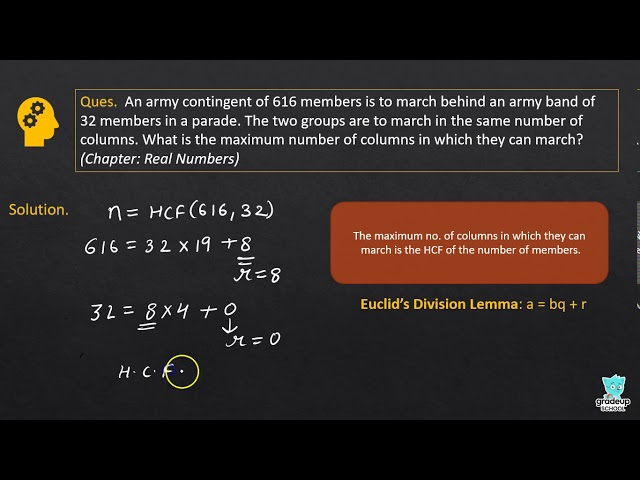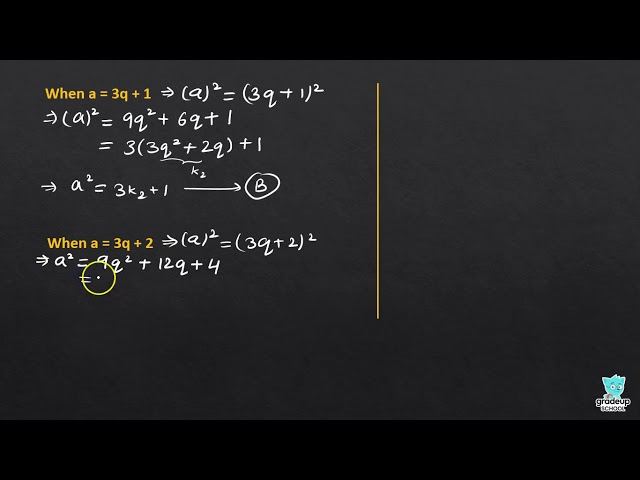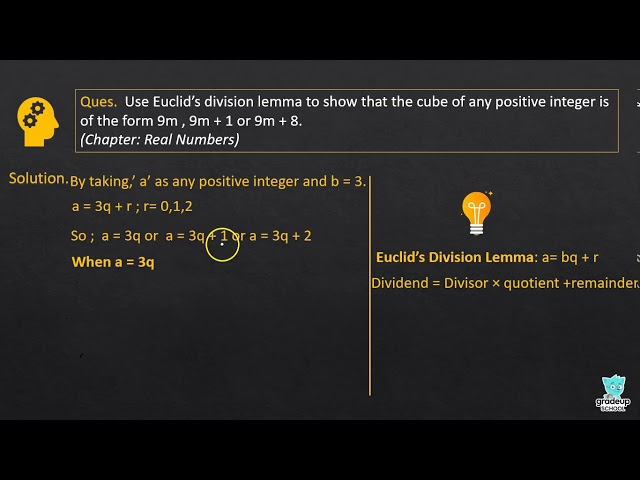NCERT - MathematicsClass 10th , NCERT
 Chapter 1 - Real Numbers Chapter 2 - Polynomials Chapter 3 - Pair of Linear Equations in Two Variables Chapter 4 - Quadratic Equations Chapter 5 - Arithmetic Progressions Chapter 6 - Triangles Chapter 7 - Coordinate Geometry Chapter 8 - Introduction to Trigonometry Chapter 9 - Some Applications of Trigonometry Chapter 10 - Circles Chapter 11 - Constructions Chapter 12 - Areas Related to Circles Chapter 13 - Surface Areas and Volumes Chapter 14 - Statistics Chapter 15 - Probability